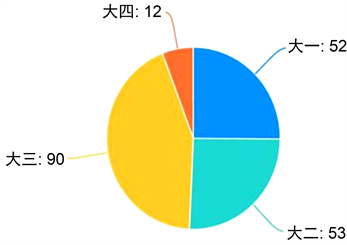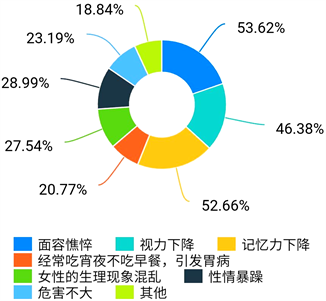# 基于Logistic模型的大学生手机依赖程度与睡眠质量的相关性分析——以曲阜师范大学统计学院为例Correlation Analysis of College Students’ Mobile Phone Dependence and Sleep Quality Based on Logistic Model—Take Statistics School of Qufu Normal University as an Example

DOI: 10.12677/SA.2020.94067, PDF, HTML, XML, 下载: 72  浏览: 1,039

Abstract: In recent years, the lack of sleep and the decline of sleep quality caused by mobile phone depend-ence have aroused widespread concern. Scientific research on the correlation between mobile phone dependence and sleep quality is related to China’s future talent training and education policy. In view of this, this paper takes the influence of mobile phone dependence on sleep quality of stu-dents in Statistics College of Qufu Normal University as an example, and analyzes the relationship between mobile phone dependence and sleep quality by using single factor analysis, t-test and F-test. Methods: The data were obtained by questionnaire. 207 students in different grades were investigated with Pittsburgh sleep quality index (PSQI), self-made questionnaire of College Students’ general situation and mobile phone dependence. At the same time, the ordinal multi classification logistic regression model combined with SPSS was used for statistical and data analysis, in order to be able to provide relevant strategies and practical reference for colleges and universities in the training of talents.

1. 引言

2. 材料与方法

2.1. 数据来源Figure 1. Sex ratioFigure 2. Proportion of students in different grades

2.2. 统计学方法

2.2.1. 手机依赖程度的判定与睡眠质量的评价准则

2.2.2. 运用SPSS建立模型并进行统计分析

3. 结果与分析

3.1. 影响大学生手机依赖的单因素分析Table 1. Single factor analysis of College Students’ mobile phone dependence ( x ¯ ± s )

3.2. 影响大学生手机依赖的多因素分析Table 2. Variable assignment method

3.2.1. 建立有序多项Logistic回归模型

$\begin{array}{l}{Y}_{0}=LOG\left[P\left(手机依赖程度=0\right)/P\left(手机依赖程度=3\right)\right]\\ \text{}=33.755-11.609年级0-9.227年级1+6.352年级2\\ \text{}-1.052家庭居住地0-1.967家庭居住地1-24.587家庭经济情况0\\ \text{}-21.862家庭经济情况1-21.870家庭经济情况2-5.079熬夜情况0\\ \text{}-0.880熬夜情况1+1.749每天使用手机情况0+0.981每天使用手机情况2\end{array}$

$\begin{array}{l}{Y}_{1}=LOG\left[P\left(手机依赖程度=0\right)/P\left(手机依赖程度=3\right)\right]\\ \text{}=36.691-17.377年级0-14.414年级1-0.396年级2-1.621家庭居住地0\\ \text{}-2.061家庭居住地1-21.219家庭经济情况0-17.684家庭经济情况1\\ \text{}-16.794家庭经济情况2-1.618熬夜情况0-0.512熬夜情况1\\ \text{}-0.326每天使用手机情况0+1.153每天使用手机情况2\end{array}$

$\begin{array}{l}{Y}_{2}=LOG\left[P\left(手机依赖程度=2\right)/P\left(手机依赖程度=3\right)\right]\\ \text{}=0.505+1.183年级0+1.354年级1-0.042年级2-0.703家庭居住地0\\ \text{}-0.104家庭居住地1-2.394家庭经济情况0+0.379家庭经济情况1\\ \text{}+0.470家庭经济情况2-1.550熬夜情况0-1.066熬夜情况1\\ \text{}-0.453每天使用手机情况0+1.164每天使用手机情况2\end{array}$

${Y}_{3}=0\left(对照组\right)$

${P}_{0}=\mathrm{exp}\left({Y}_{0}\right)/\left[\mathrm{exp}\left({Y}_{0}\right)+\mathrm{exp}\left({Y}_{1}\right)+\mathrm{exp}\left({Y}_{2}\right)+\mathrm{exp}\left({Y}_{3}\right)\right]$

${P}_{1}=\mathrm{exp}\left({Y}_{1}\right)/\left[\mathrm{exp}\left({Y}_{0}\right)+\mathrm{exp}\left({Y}_{1}\right)+\mathrm{exp}\left({Y}_{2}\right)+\mathrm{exp}\left({Y}_{3}\right)\right]$

${P}_{2}=\mathrm{exp}\left({Y}_{2}\right)/\left[\mathrm{exp}\left({Y}_{0}\right)+\mathrm{exp}\left({Y}_{1}\right)+\mathrm{exp}\left({Y}_{2}\right)+\mathrm{exp}\left({Y}_{3}\right)\right]$

${P}_{3}=\mathrm{exp}\left({Y}_{3}\right)/\left[\mathrm{exp}\left({Y}_{0}\right)+\mathrm{exp}\left({Y}_{1}\right)+\mathrm{exp}\left({Y}_{2}\right)+\mathrm{exp}\left({Y}_{3}\right)\right]$

3.2.2. Logistic模型的检验Table 3. Model fitting informationTable 6. Likelihood ratio test table

a. 因为省略此效应并不会增加自由度，所以此简化模型相当于最终模型。

3.3. 不同手机依赖程度对睡眠质量的影响Table 7. Comparison of total PSQI scores of college students with different mobile phone dependence

3.4. 手机依赖与睡眠质量各因子相关性

PSQI量表中，将睡眠质量分为7个因子，采用SPSS分析，结果表明7个因子与手机依赖总分及其各维度均呈正相关，见表8Table 8. Correlation coefficient between mobile phone dependence and sleep quality of College Students

3.5. 分析

3.5.1. 手机依赖因素分析

3.5.2. 手机依赖与睡眠质量相关性分析

4. 讨论

4.1. 结论Figure 3. Mental state on the second day after staying up lateFigure 4. Changes in body due to staying up late

4.2. 建议

4.2.1. 正确看待，将手机视为双刃剑

4.2.2. 打造良好环境，营造健康手机使用氛围

4.2.3. 积极开发基于手机平台的学习方式，有效促进学生学习

4.2.4. 教育工作者采取措施，引导大学生合理使用手机

  李娅凌, 杨超, 张俊辉, 等. 某医学院学生睡眠质量现状及影响因素的Logistic回归分析[J]. 泸州医学院学报, 2015(2): 145-149.  张红, 陈元金, 杨玉莹. 大学生手机依赖程度与睡眠质量的相关性分析[N]. 当代护士(上旬刊), 2019, 26(5): 9-11.  山雨琴, 林亚玲, 吴俊辰, 等. 基于多分类Logistic睡眠质量影响指标的研究[J]. 科技创新导报, 2018(8): 253-254, 256.  江燕, 朱家明. 基于logistic回归对失眠和疾病关系的研究[J]. 哈尔滨师范大学自然科学学报, 2018, 34(1): 8-14.  吴继辉, 杨华磊. 大学生睡前玩智能手机对睡眠质量的影响分析[A]. 管理观察, 2016, 614: 85-87.